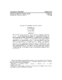## Analysis of the Residual Arnoldi MethodThe Arnoldi method generates a nested squences of orthonormal bases $U_{1},U_{2}, \ldots$ by orthonormalizing $Au_{k}$ against $U_{k}$. Frequently these bases contain increasingly accurate approximations of eigenparis from the periphery of the spectrum of $A$. However, the convergence of these approximations stagnates if $u_{k}$ is contaminated by error. It has been observed that if one chooses a Rayleigh--Ritz approximation $(\mu_{k}, z_{k})$ to a chosen target eigenpair $(\lambda, x)$ and orthonormalizes the residual $Az_{k - }\mu_{k} z_{k}$, the approximations to $x$ (but not the other eigenvectors) continue to converge, even when the residual is contaminated by error. The same is true of the shift-invert variant of Arnoldi's method. In this paper we give a mathematical analysis of these new methods.